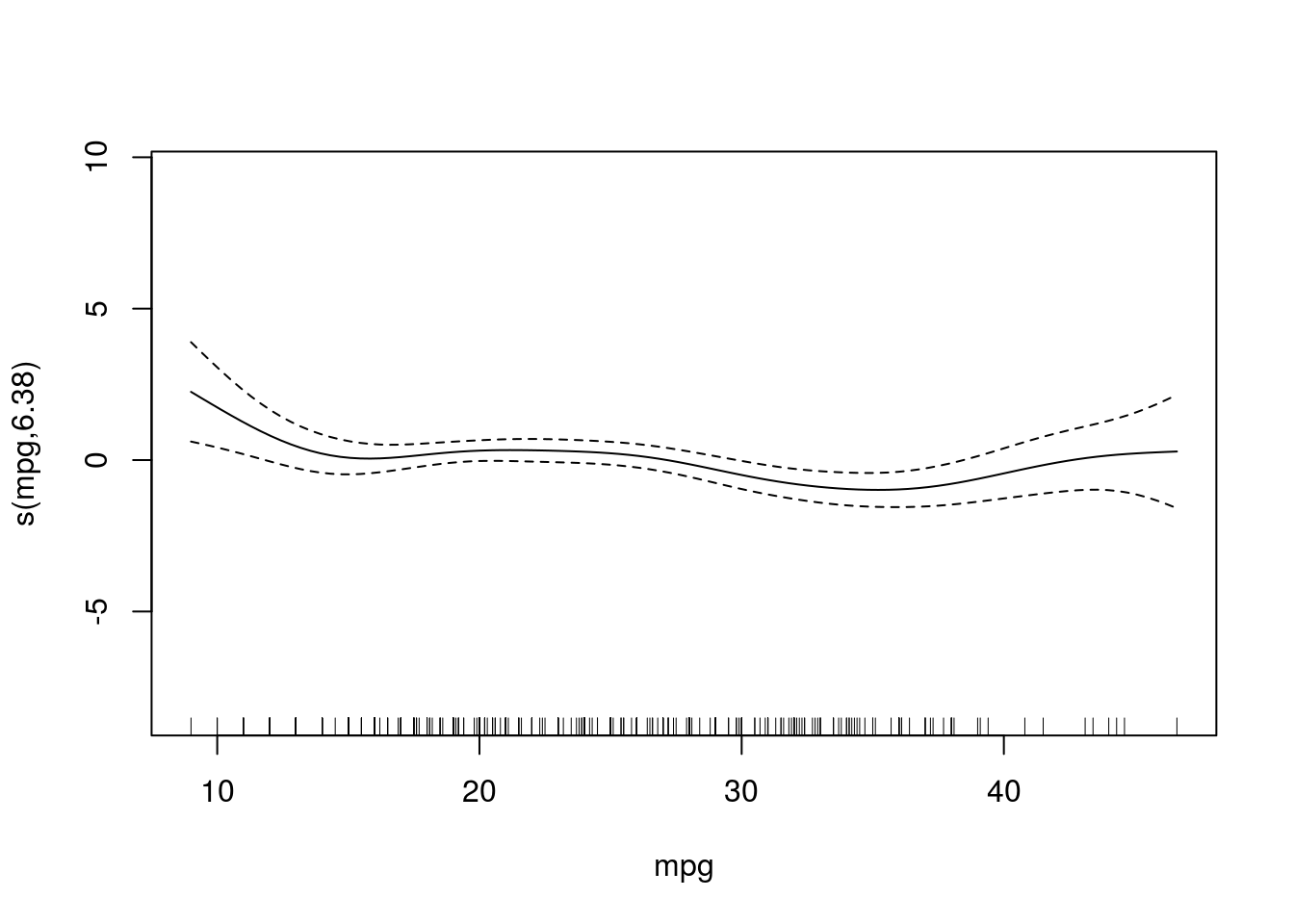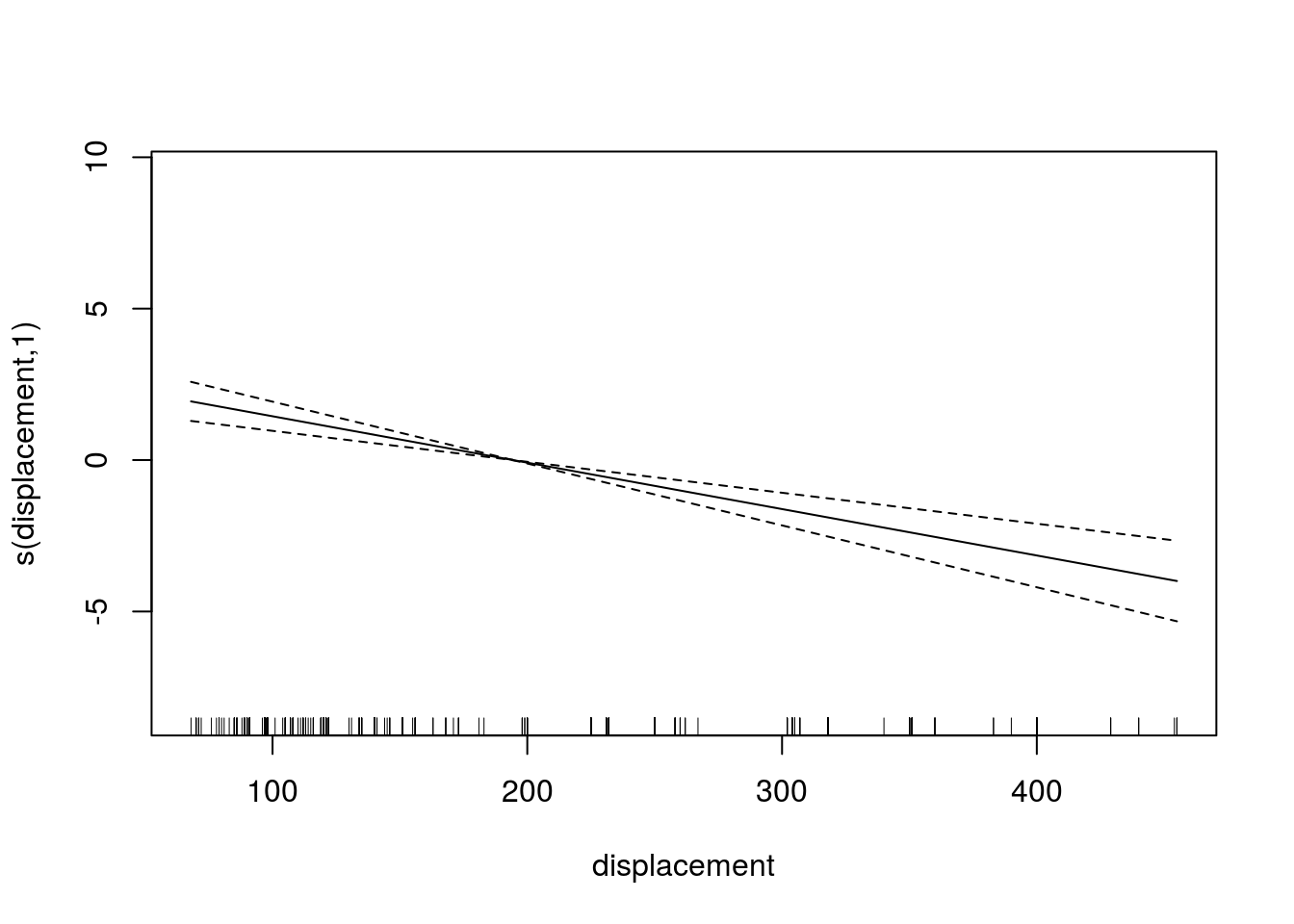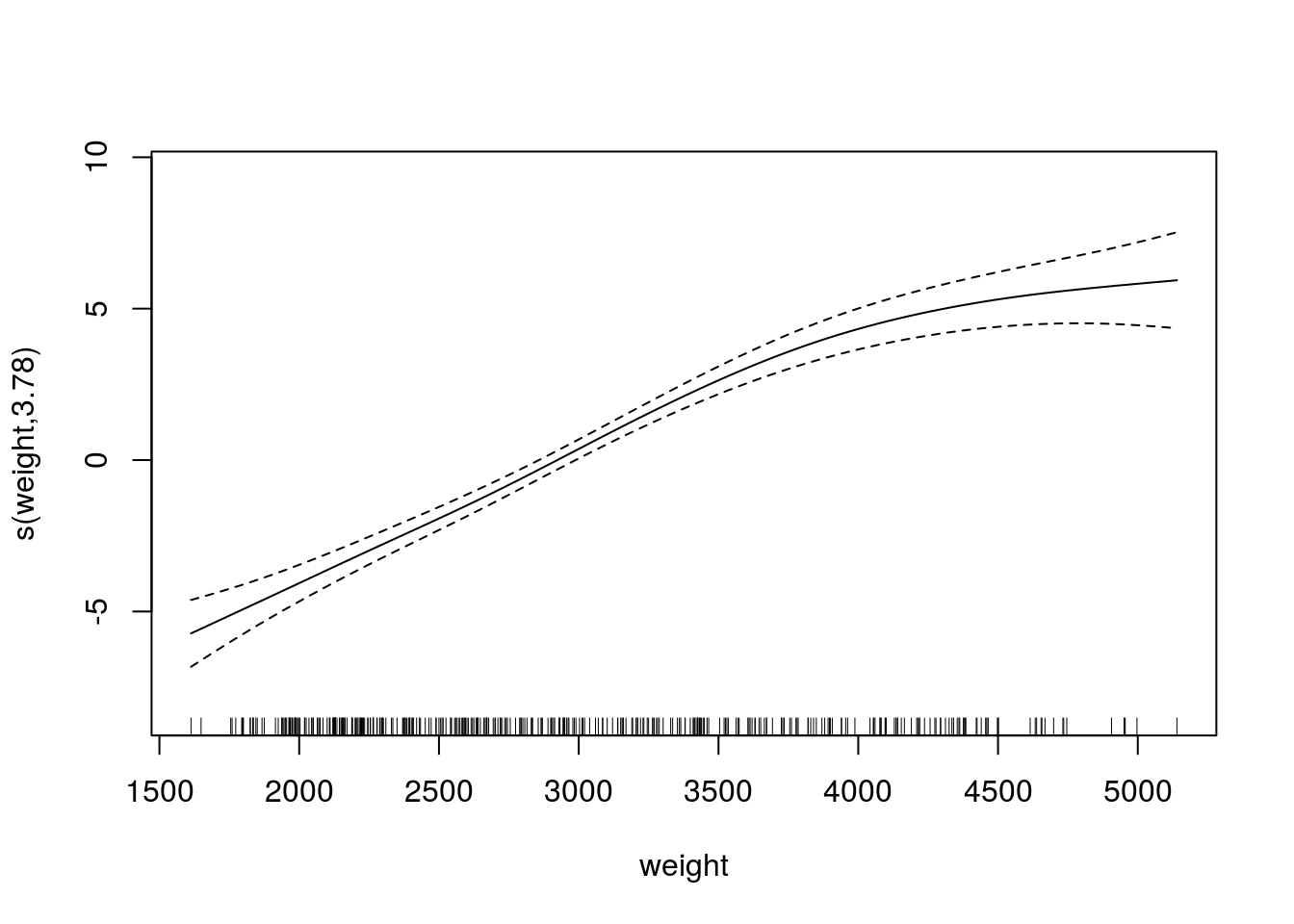# Generalized Additive Models in R

In this post, we will learn how to create a generalized additive model (GAM). GAMs are non-parametric generalized linear models. This means that linear predictor of the model uses smooth functions onthe predictor variables. As such, you do not need to specify the functional relationship between the response and continuous variables. This allows you to explore the data for potential relationships that can be more rigorously tested with other statistical models

In our example, we will use the “Auto” dataset from the “ISLR” package and use the variables “mpg”,“displacement”,“horsepower”, and “weight” to predict “acceleration”. We will also use the “mgcv” package. Below is some initial code to begin the analysis

library(mgcv)
library(ISLR)
data(Auto)

We will now make the model we want to understand the response of “acceleration” to the explanatory variables of “mpg”,“displacement”,“horsepower”, and “weight”. After setting the model we will examine the summary. Below is the code

model1<-gam(acceleration~s(mpg)+s(displacement)+s(horsepower)+s(weight),data=Auto)
summary(model1)
##
## Family: gaussian
##
## Formula:
## acceleration ~ s(mpg) + s(displacement) + s(horsepower) + s(weight)
##
## Parametric coefficients:
##             Estimate Std. Error t value Pr(>|t|)
## (Intercept) 15.54133    0.07205   215.7   <2e-16 ***
## ---
## Signif. codes:  0 '***' 0.001 '**' 0.01 '*' 0.05 '.' 0.1 ' ' 1
##
## Approximate significance of smooth terms:
##                   edf Ref.df      F  p-value
## s(mpg)          6.382  7.515  3.479  0.00101 **
## s(displacement) 1.000  1.000 36.055 4.35e-09 ***
## s(horsepower)   4.883  6.006 70.187  < 2e-16 ***
## s(weight)       3.785  4.800 41.135  < 2e-16 ***
## ---
## Signif. codes:  0 '***' 0.001 '**' 0.01 '*' 0.05 '.' 0.1 ' ' 1
##
## R-sq.(adj) =  0.733   Deviance explained = 74.4%
## GCV = 2.1276  Scale est. = 2.0351    n = 392

All of the explanatory variables are significant and the adjust r-squared is .73 which is excellent. edf stands for “effective degrees of freedom”. This modified version of the degree of freedoms is due to the smoothing process in the model. GCV stands for generalized cross-validation and this number is useful when comparing models. The model with the lowest number is the better model.

We can also examine the model visually by using the “plot” function. This will allow us to examine if the curvature fitted by the smoothing process was useful or not for each variable. Below is the code.

plot(model1)We can also look at a 3d graph that includes the linear predictor as well as the two strongest predictors. This is done with the “vis.gam” function. Below is the code

vis.gam(model1)If multiple models are developed. You can compare the GCV values to determine which model is the best. In addition, another way to compare models is with the “AIC” function. In the code below, we will create an additional model that includes “year” compare the GCV scores and calculate the AIC. Below is the code.

model2<-gam(acceleration~s(mpg)+s(displacement)+s(horsepower)+s(weight)+s(year),data=Auto)
summary(model2)
##
## Family: gaussian
##
## Formula:
## acceleration ~ s(mpg) + s(displacement) + s(horsepower) + s(weight) +
##     s(year)
##
## Parametric coefficients:
##             Estimate Std. Error t value Pr(>|t|)
## (Intercept) 15.54133    0.07203   215.8   <2e-16 ***
## ---
## Signif. codes:  0 '***' 0.001 '**' 0.01 '*' 0.05 '.' 0.1 ' ' 1
##
## Approximate significance of smooth terms:
##                   edf Ref.df      F p-value
## s(mpg)          5.578  6.726  2.749  0.0106 *
## s(displacement) 2.251  2.870 13.757 3.5e-08 ***
## s(horsepower)   4.936  6.054 66.476 < 2e-16 ***
## s(weight)       3.444  4.397 34.441 < 2e-16 ***
## s(year)         1.682  2.096  0.543  0.6064
## ---
## Signif. codes:  0 '***' 0.001 '**' 0.01 '*' 0.05 '.' 0.1 ' ' 1
##
## R-sq.(adj) =  0.733   Deviance explained = 74.5%
## GCV = 2.1368  Scale est. = 2.0338    n = 392
#model1 GCV
model1$gcv.ubre ## GCV.Cp ## 2.127589 #model2 GCV model2$gcv.ubre
##   GCV.Cp
## 2.136797

As you can see, the second model has a higher GCV score when compared to the first model. This indicates that the first model is a better choice. This makes sense because in the second model the variable “year” is not significant. To confirm this we will calculate the AIC scores using the AIC function.

AIC(model1,model2)
##              df      AIC
## model1 18.04952 1409.640
## model2 19.89068 1411.156

Again, you can see that model1 s better due to its fewer degrees of freedom and slightly lower AIC score.

Conclusion

Using GAMs is most common for exploring potential relationships in your data. This is stated because they are difficult to interpret and to try and summarize. Therefore, it is normally better to develop a generalized linear model over a GAM due to the difficulty in understanding what the data is trying to tell you when using GAMs.